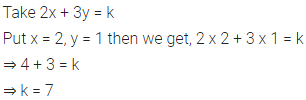# Find the value of k, if x = 2, y = 1 is a solution of the equation 2x + 3 y = k

Find the value of k, if x = 2, y = 1 is a solution of the equation 2x + 3 y = k.